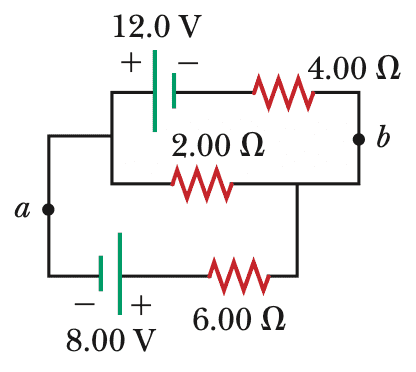# Finding the potential difference in a circuit

jolly_math
Homework Statement:
For the circuit below, calculate the potential difference between points a and b. The current in the 2.00 Ω resistor is 0.909 A, and the current in the 4.00 Ω resistor is 1.636 A.
Relevant Equations:
loop rule
junction ruleThe solution chooses the centre wire to determine the potential difference, where Va−(0.909 A)(2.00 Ω)=Vb and Vb - Va = -1.82

If I choose the top wire (passing through the 12 V battery and 4 Ω resistor), Va - 12 + (1.636 A)(4.00 Ω)=Vb, and Vb - Va is different (= -5.46 V). Why would this path not work?

Thank you.

Homework Helper
Gold Member
I think the problem is that the situation they describe is impossible, as it breaks Kirchhoff's circuit law for voltage. Given the currents they've specified for the top two resistors, the PDs across the three elements in the upper circuit are 12, 0.909 x 2 and 1.636 x 4 and, whatever signs we give to either of those last two, we cannot get the three PDs to add to zero as Kirchhoff requires.

I suspect the question just contains a misprint, which makes it unsolvable.

For an impossible circuit, we should not be surprised if it gives different measurements when approached in different ways.

EDIT: In fact, they should not specify any currents in the circuit. We can calculate all currents using just the voltages of the two cells and the three resistances. Use Kirchhoff's laws. The current they specify for the 2 Ohm resistor is correct but that for the 4 Ohm resistor is not. It needs to be way more than that.

Last edited:
•jolly_math, phinds, TSny and 1 other person
Homework Helper
Gold Member
The current they specify for the 2 Ohm resistor is correct but that for the 4 Ohm resistor is not.
Yes. The 1.636 A is the current in the 6 Ohm resistor.

•SammyS and andrewkirk
jolly_math
I think the problem is that the situation they describe is impossible, as it breaks Kirchhoff's circuit law for voltage. Given the currents they've specified for the top two resistors, the PDs across the three elements in the upper circuit are 12, 0.909 x 2 and 1.636 x 4 and, whatever signs we give to either of those last two, we cannot get the three PDs to add to zero as Kirchhoff requires.

I suspect the question just contains a misprint, which makes it unsolvable.

For an impossible circuit, we should not be surprised if it gives different measurements when approached in different ways.

EDIT: In fact, they should not specify any currents in the circuit. We can calculate all currents using just the voltages of the two cells and the three resistances. Use Kirchhoff's laws. The current they specify for the 2 Ohm resistor is correct but that for the 4 Ohm resistor is not. It needs to be way more than that.
Okay, thank you. Ignoring the actual values, could either path be used to get the same potential difference?

•